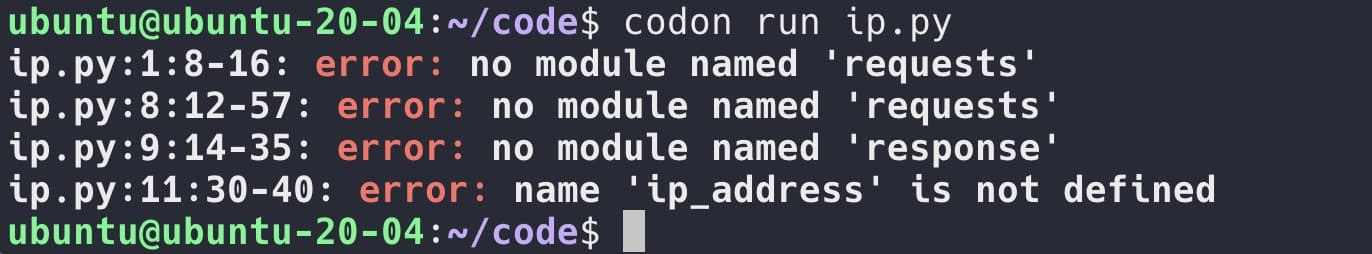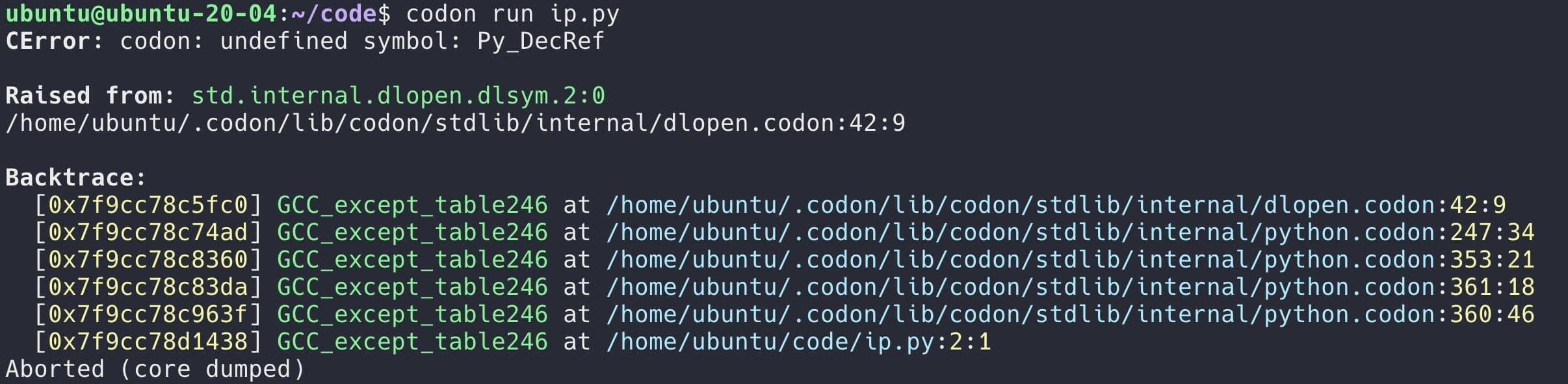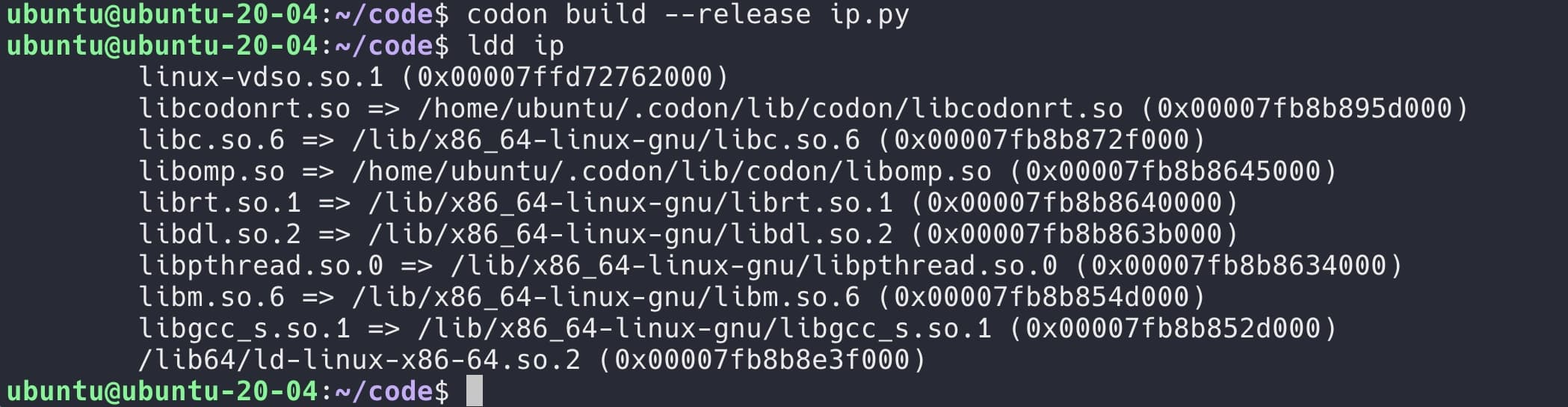# 使用 Codon 提升 Python 代码执行速度

Published: 2023-03-22

Tags: Python

Codon 是一款高性能的 Python 编译器，它可以将 Python 代码编译成本地机器代码，不会产生任何运行时开销。通常情况下，相对于 Python，单线程的速度提升可以达到 100 倍或更多，Codon 支持本地多线程，这可能会极大的提升执行速度。

### Quick Start

``````from time import time

def fib(n):
return n if n < 2 else fib(n - 1) + fib(n - 2)

t0 = time()
ans = fib(36)
t1 = time()
print(f'Computed fib(36) = {ans} in {t1 - t0} seconds.')
``````### 提升特定函数性能

``````\$ pip3 install codon-jit
``````

``````import codon
from time import time

def is_prime_python(n):
if n <= 1:
return False
for i in range(2, n):
if n % i == 0:
return False
return True

@codon.jit
def is_prime_codon(n):
if n <= 1:
return False
for i in range(2, n):
if n % i == 0:
return False
return True

t0 = time()
ans = sum(1 for i in range(100000, 110000) if is_prime_python(i))
t1 = time()
print(f'[python] {ans} | took {t1 - t0} seconds')

t0 = time()
ans = sum(1 for i in range(100000, 110000) if is_prime_codon(i))
t1 = time()
print(f'[codon]  {ans} | took {t1 - t0} seconds')
``````### 使用三方模块示例

``````import requests

'User-Agent': 'curl/7.68.0',
'Accept': '*/*'
}

```````import requests` 替换为 `from python import requests```````# ubuntu
export CODON_PYTHON=/usr/lib/x86_64-linux-gnu/libpython3.10.so

# centos
export CODON_PYTHON=/usr/lib64/libpython3.6m.so
``````• `from python import`
• `@python`

### 关于 Build 可行性文件### Codon 的文档

Codon 可以执行绝大部分的 Python 原生代码，Codon 也有着自己的语法，更多的差异与特性，参考官方文档：